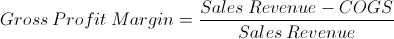# Gross Profit MarginGross profit margin is a profitability ratio that is found by dividing a company's gross profit by its revenues. Gross profit is a company's revenues minus the COGS, Cost of Goods Sold. The cost of goods sold, or cost of sales, and sales revenues are both found on a company's income statement.

The gross margin is often used with other profitability ratios to evaluate how much a company's sales will result in earnings, after covering the company's expenses. The gross margin, specifically, looks at the direct cost of the goods or services offered by the company. For comparison, the operating margin looks at a company's earnings after subtracting the COGS and operating expenses. Operating expenses are general business expenses that are unrelated to the direct cost of production. Also, for comparison, the net profit margin looks at net income, which is earnings after COGS, operating expenses, and interest and tax expenses. In other words, the net margin looks at earnings after all expenses are accounted for. All of the margin ratios explained here are stated in relation to total revenues, just like the gross profit margin formula at the top of the page.

## Example of Gross Profit Margin Formula

Assume that a company is reviewing their production costs. The recent income statement shows revenues of \$20mil and Cost of Goods Sold of \$10mil. For the sake of simplicity, round numbers are used. Using the gross profit margin formula at the top of the page, the numerator, gross profit, is \$20mil minus \$10mil. This results in a gross profit of \$10mil.

From here, we can divide the \$10mil gross profit by \$20mil revenues to get a gross margin of 50%. As stated earlier, there are additional business expenses and other profitability ratios, however this formula can help review the actual production process.

## Use of Gross Profit Margin Formula

The gross profit margin formula, together with the other profit margin formulas, can be used by companies to compare the company's ability to turn a profit beyond its expenses. By putting the income in relative terms, in relation to expenses, a company can determine how well it is maintaining costs. The gross profit margin, specifically, looks at how much a company is earning after accounting for its production costs.

Investors may also use this formula for the same reasons as companies do, but for the sake of comparing different investment opportunities. For this formula specifically, it is important to compare like companies. Different industries often will have different margins.

New to Finance?